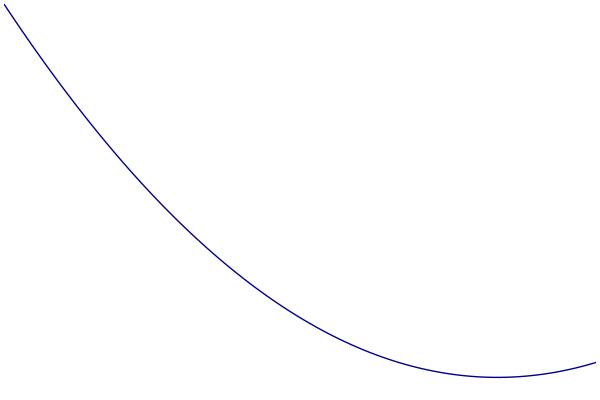# MM Algorithms

## Definition

Majorization-Minimization (MM) is an important concept in optimization. It is more of a principle than a specific algorithm, and many algorithms can be interpreted under the MM framework (coordinate descent, proximal gradient method, EM Algorithm, etc.). The main idea is the concept of a majorizing function.

A Function $h(\theta)$ is said to majorize $f(\theta)$ at $\theta^{(t)}$ if

\begin{aligned} h(\theta | \theta^{(t)}) &\ge f(\theta | \theta^{(t)}) \quad\text{(dominance condition)}, \\ h(\theta^{(t)} | \theta^{(t)}) &= f(\theta^{(t)} | \theta^{(t)}) \quad\text{(tangent condition)}. \end{aligned}

Simply put, the surface of $h(\theta)$ lies above $f(\theta)$, apart from at $\theta^{(t)}$ where they are equal. An MM update consists of minimizing the majorization:

$\theta^{(t+1)} = \text{argmin}_\theta \;h(\theta|\theta^{(t)}).$

Things to note:

• MM updates are guaranteed to decrease the value of the objective function (descent property).

• Majorizations are often used to split parameters, allowing updates to be done element-wise.

## Visualization

• Blue: Objective

• Green: Majorization

• Red: Parameter Estimate## Example: Logistic Regression

### Majorization

One technique for majorizing a convex function is a quadratic upper bound. If we can find a matrix $M$ such that $M - d^2f(\theta)$ is nonnegative definite for all $\theta$, then

$f(\theta) \le f(\theta^{(t)}) + \nabla f(\theta^{(t)})^T(\theta - \theta^{(t)}) + \frac{1}{2}(\theta - \theta^{(t)})^T M (\theta - \theta^{(t)}).$

Using the RHS as our majorization, updates take the form

$\theta^{(t+1)} = \theta^{(t)} - M^{-1}\nabla f(\theta^{(t)}).$

This method produces an update rule that looks like Newton's method, but uses an approximation of the Hessian which guarantees descent.

### Logistic Regression

The negative log-likelihood for the logistic regression model provides the components

\begin{aligned} f(\beta) &= \sum_i \left\{-y_i x_i^T\beta + \ln\left[1 + \exp(x_i^T\beta)\right]\right\} \\ \nabla f(\beta) &= \sum_i -[y_i - \hat{y_i}(\beta)]x_i \\ d^2 f(\beta) &= \sum_i \hat{y_i}(\beta)[1 - \hat{y_i}(\beta)]x_i x_i^T \end{aligned}

where each $x_i$ is a vector of predictors, $y_i\in\{0, 1\}$ is the response, and $\hat{y_i}(\beta) = (1 + \exp(-x_i^T\beta))^{-1}$. Continuing to create the majorization:

• In matrix form, the Hessian is $d^2 f(\beta) = X^TWX$, where $W$ is a diagonal matrix with entries $\hat{y_i}(1 - \hat{y_i})$.

• $\hat{y_i}$ can only take values in (0, 1), so $\frac{1}{4} \ge \hat{y_i}(1 - \hat{y_i})$.

• Therefore, we can use $M = X^TX/4$ to create a quadratic upper bound. Compare this MM update to Newton's method.

$\beta^{(t+1)} = \beta^{(t)} - 4\left(X^TX\right)^{-1}X^T(y - \hat{y}(\beta^{(t)}))$
$\beta^{(t+1)} = \beta^{(t)} - \left(X^TWX\right)^{-1}X^T(y - \hat{y}(\beta^{(t)}))$
In this case, MM has the advantage of being able to reuse $(X^TX)^{-1}$ after it is computed once, whereas Newton's method must solve a linear system at each iteration.